Smartick is a fun way to learn math!Aug31

In this post, we are going to look at a numerical and logic puzzle. It is very similar to Sudoku, as it requires mathematical and problem-solving skills. If you want, you can review Sudoku: What is it, and how is it solved? Now, let’s learn more about number puzzles!

Index

## What is this number puzzle?

It is a fun and educational mental challenge invented by Japanese mathematician Tetsuya Miyamoto in 2004. The puzzle consists of a grid of cells, just like Sudoku, but the cells are not divided into smaller regions but, instead, some cells are grouped and highlighted. They can be highlighted using a thick border or grouped by color and contain a number along with a mathematical symbol (+, -, ×, ÷). These groups of cells are called “cages”, and here at Smartick a cage is formed by the cells that have the same color.

The aim of the game is to complete each cell with a number from 1 to n (where n is the size of the grid) so that the following conditions are met:

• Each number must appear only once in each row and in each column, just as in Sudoku.
• The numbers in each cage must be combined using the operation indicated in the cage (addition, subtraction, multiplication or division) to obtain the indicated result.
• Numbers within a cage cannot be repeated.

## How do you solve this number puzzle?

To solve this type of number puzzle, we have to take into account the three rules mentioned above. Let’s look at an example of a number puzzle from a Smartick session!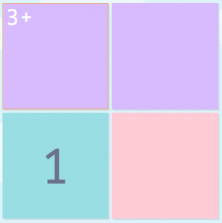1. The first thing we have to do is to look at the dimension of the puzzle to know which numbers we need to fill in the cells. In this case, the number puzzle is 2×2, so we have to use the numbers 1 and 2.
2. There are different colored regions, those are the cages! You have to complete them little by little. In this example, what colors are the cages and how many are there? Got the answer? There are 3 cages:
1. A purple cage
2. A blue cage
3. A pink cage
3. Now, we are going to complete the colored cages. Let’s start with the purple cage. We need to check if there is a number in the upper left corner of the cells. It says 3+! That is the exact number you would get after performing the indicated operation (addition, subtraction, multiplication or division). So, if we can only use the numbers 1 and 2 and the cells of that cage must add up to 3, we have to place a 1 and a 2, but where do we place each one? Since in the first column there is already a 1 in the blue cage, and the rules tell us that no number can be repeated in a row or in a column, in the top cell there can only be a 2. As a result, in the other cell of the purple cage, there will be a 1.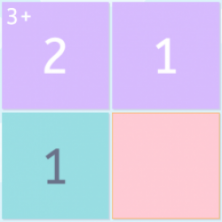4. Finally, we have to fill in the pink cage, which consists of only one cell. What number will go here? Just as before, we cannot repeat numbers in the same row or in the same column. Therefore, we can only write a 2.
5. The number puzzle would therefore look like this: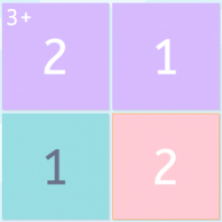## Strategies for solving difficult number puzzles

• Step 1: Understand the basic rules.
Imagine you are completing a grid with numbers. Each row and column must have all numbers from 1 to the number of cells in the grid, without repeating any numbers.
• Step 2: Look at the large cages.
Start by looking at the largest cells. Each cell has a number and a sign (+, -, ×, ÷). You have to combine those numbers based on the sign of the operation so that the number in the corner is the result. Use this to fill in some cells to help complete the puzzle.
• Step 3: Find unique cells.
Look for rows and columns that have only one empty space. You must put the missing number in that cell. Make sure this number is not in the same row or column.
• Step 4: Use numbers you already know.
If you have already placed a number in a cell, think about how it affects nearby cells. Some cells may only have certain numbers because of this.
• Step 5: Test and see.
If you are not sure, try placing different numbers in the cells to see what works. But be sure to try combinations that make sense. If something doesn’t fit, delete and try again.
• Step 6: Have fun solving!
Solve the puzzle one step at a time. Don’t worry if it takes time. Bit by bit, you’ll find the pattern and figure out the best way to place the numbers – don’t give up and have fun figuring it out!

## Let’s practice

Number puzzle 1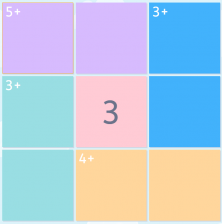Number puzzle 2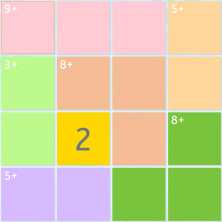### Solutions

Number puzzle 1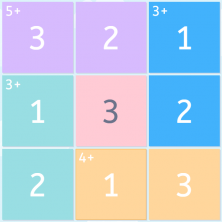Number puzzle 2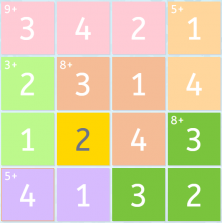Remember, practice makes everything easier! Over time, you will become better at solving these puzzles. Good luck and enjoy solving them!

Anyone with different levels of mathematical ability can solve these types of number puzzles, as there are puzzles of different sizes and levels of difficulty available. Smartick’s logic sessions follows a sequence in which you will work on solving these puzzles, starting with the easiest examples, moving on to others of greater difficulty. Check it out, sign up and try the Smartick method for free!